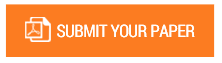Construction of a Positive Valued Scalar Function of Strictly Rectangular Complex Matrices using the Framework of Spacer Matrix Components and Related Matrices

Authors : Debopam Ghosh

Volume/Issue : Volume 7 - 2022, Issue 12 - December

Scribd : https://bit.ly/3j7iJvZ

DOI : https://doi.org/10.5281/zenodo.7445012

The Research paper presents a mathematical method to construct a real, positive valued scalar function of matrices belonging to the strictly rectangular complex matrix spaces. The formulated method leads to association of a strictly rectangular complex matrix with a positive vector whose components sum to unity and belongs to the real co-ordinate space of dimensionality equal to that of the embedding dimension associated with the input degrees of freedom of the rectangular complex matrix space. These vector components act as the respective weights to the ordered eigenvalues (ordered from largest to smallest) of a real, hermitian and positive definite matrix, termed as the Reference matrix, which is uniquely determined by the Spacer component matrices associated with the strictly rectangular complex matrix space. The resulting weighted sum of these eigenvalues, expressed as an appropriate Inner product, is the numerical value which the scalar function assigns as the output for the input complex strictly rectangular matrix under consideration.

Keywords : Spacer matrix based generalized matrix multiplication, Spacer matrix components, Embedding

#### CALL FOR PAPERS

Paper Submission Last Date
31 - March - 2023